#### How to Do Regression Analysis in Excel

Regression analysis is a statistical tool which is used for analyzing and modeling variables, it lets you see how value of one variable (dependent) changes when any one of the other independent variables changes. It helps us to understand the relationship between a dependent variable and one or more independent variables

In other words Regression is a method of estimating the portion of a cost that is variable and the portion that is fixed. The following tutorial will help you use Excel to “run” a regression.

## Accessing Regression Analysis in Microsoft Excel

Go to File > Excel Options > Add-Ins > click Analysis ToolPak > then click GO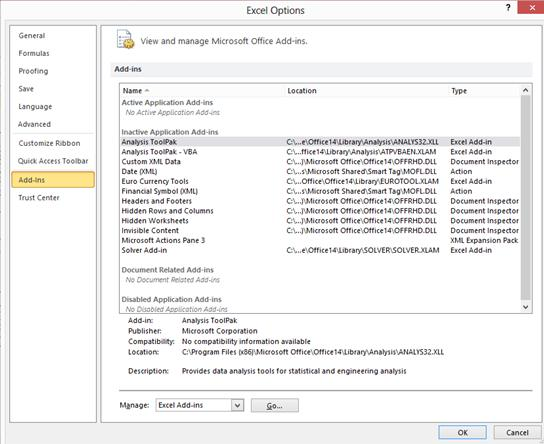Following dialog box will be open, Click Analysis Toolpak and then click OK.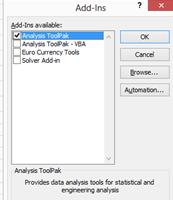### Data analysis

Data Analysis button will appear on Data ribbon. Under Data Analysis feature Regression function can be found.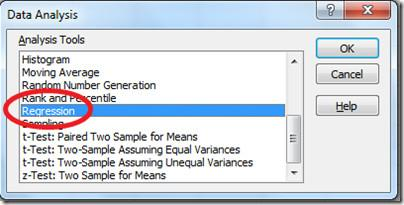Once Data Analysis is enabled, open it and choose the Regression and click ok button.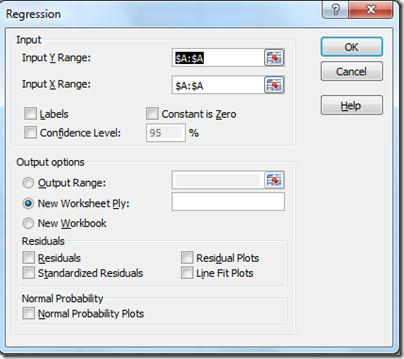• Input Y Range option: Select dependent variables
• Input X Range option: Select independent variables.
• Output options specify how you would like the results to be displayed,
• Residuals contain options to draw the results as charts.

For practice type X & Y values in the following style: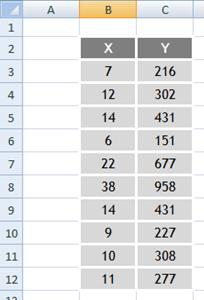### Summary Output

Once you click the Ok button following regression table will be created in new sheet as follows.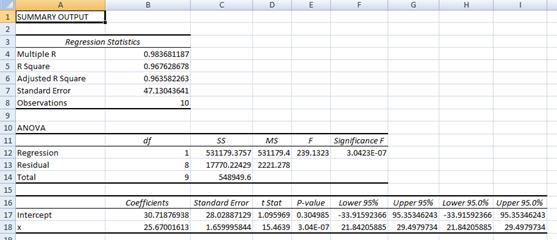The only thing which left is to analyze the summary output.

## Linear Regression in Excel

The linear regression is similar to multiple regression. But, there are difference between them.

### How it works?

The linear regression function answers the question: “What value will a given phenomenon (dependent variable) take, depending on the value of another phenomenon (explanatory variable)?” Due to the term “linear”, a method which has a way to remove the explained phenomenon and the variable explanation is precisely linear.

### How to calculate?

We’d perform the task that together, in a step-by-step format. But, first you’d need to get the Data Analysis by following through these steps: file > options > add-ins> go > data analysis > ok. Further, you’d also needs a data that look like this: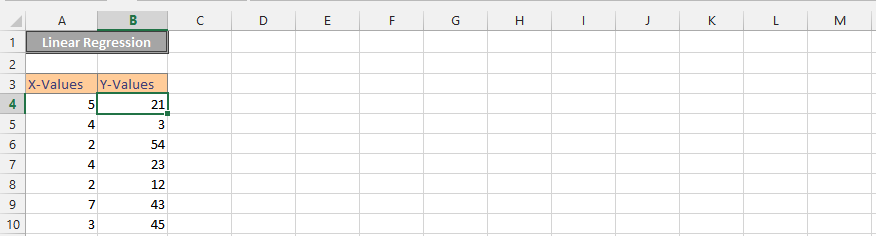1. Click on Data (1), and then press on the Data Analysis (2).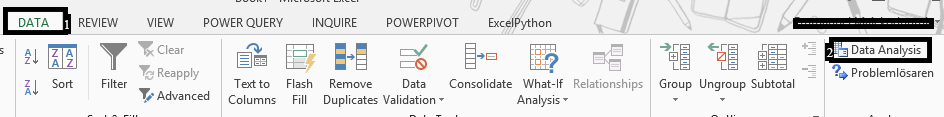2. Click on the regression.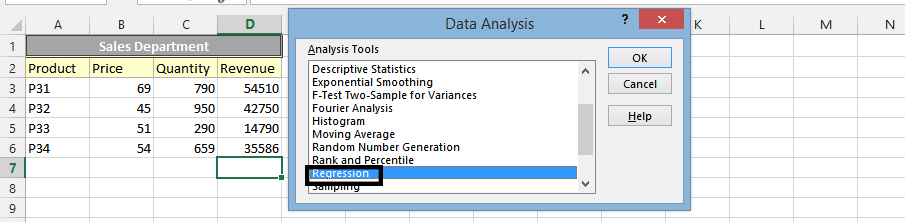3. Select the cells for y-values (1), then the ones for x-values (2), and then check the labels (3). Finally, press ok (4).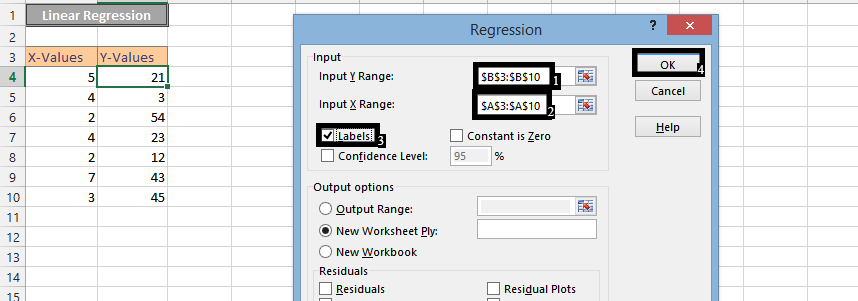4. Click on an empty cell (1), and then press insert (2). Press scatter chart (3), and choose a scatter chart (4).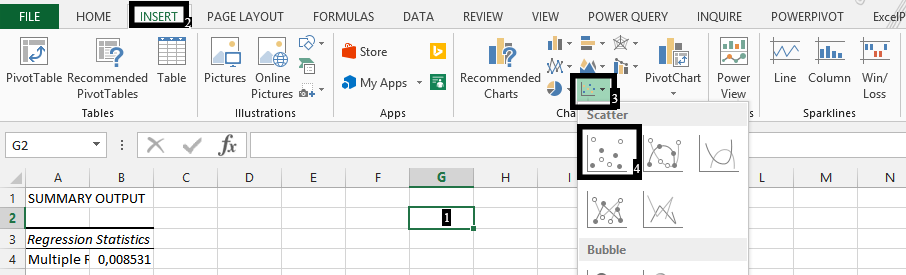5. Right click on the chart (1), and choose Select Data (2).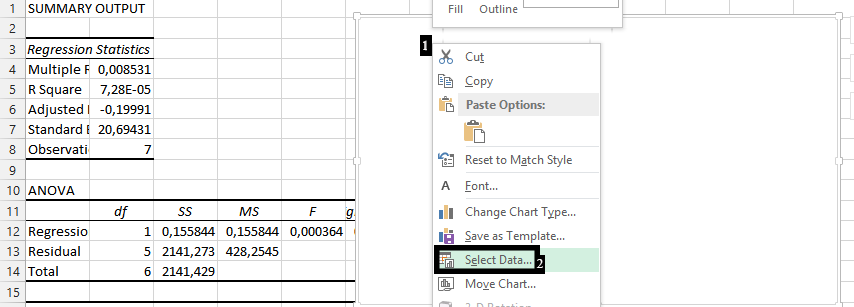7. Write the series name (1), choose the cells for X-values (2), and for Y-values (3). Press ok (4).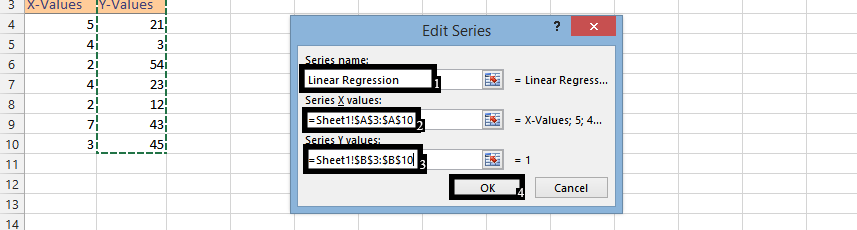Note: The values are the data, which would be in another spreadsheet.

8. Click Ok.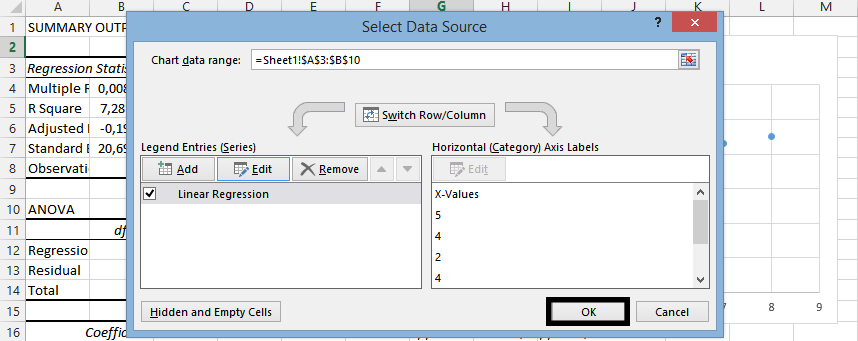9. Right Click on any of the markers (1), and then select add trendline (2).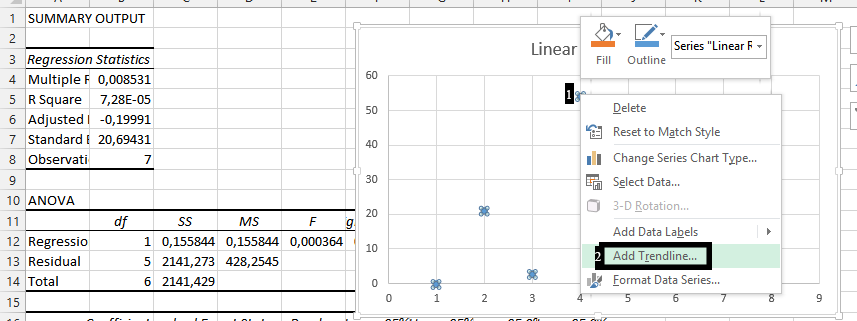10. If you would prefer, check the Display Equation on Chart (1), and the R-square value on chart (2).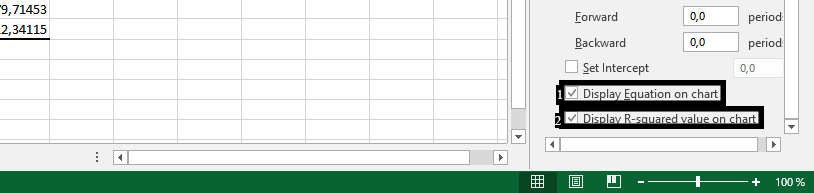You should have done a linear regression that looks like this: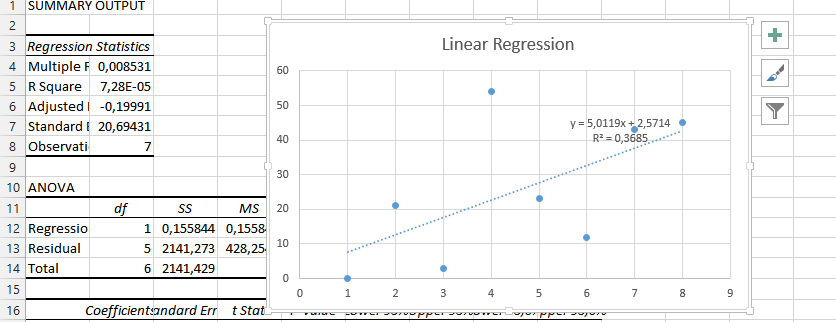## Multiple Regression in Excel

The multiple regression is the way how to check the relationship of two features.

In order for the model to be as reliable as possible, the largest possible number of independent variables should be introduced into the model. The model should contain variables strongly correlated with the dependent variable and at the same time as weakly correlated with each other as possible.

### Examples of multiple regression

• The volume of sales depends on the proportion in which the advertising campaign was carried out in three different local newspapers. Question: What is the proportion of spending on an advertising campaign in these newspapers so that the sale is the highest?
• The appraisal of an apartment depends on many characteristics of the property, such as: area, number of rooms, age, type of heating, district, number of floors. From the developer’s point of view, the analysis will show the value of the apartment he is going to build. Maybe it is worth adding a garage?
• The bank wants to check whether it should grant the customer a loan. He knows his income, education, age, savings, marital status, number of children. Based on the analysis of these features, the bank wants to receive data on the risk that the customer will not repay the loan. If a high risk of loan default is identified, the bank will refuse to grant a loan based on a regression analysis.
• A good example is also the study of life expectancy based on factors such as: neighborhood, health condition, medications taken, physical activity, etc.

### How to calculate?

The multiple regression in Microsoft Excel is possible. The best method is a step-by-step instruction that will show you how. The first thing is having a data that could look something like this:1. Click on data (1), and then click on the data analysis (2)Note: The data analysis could be gotten from the add-in (file > options > add-ins > Go).

2. Choose Regression in the Data Analysis, and click ok.3. Choose the data you’d want to analyze on X values (1), and Y values (2). Select desired options, under the residuals (3), and press ok (4).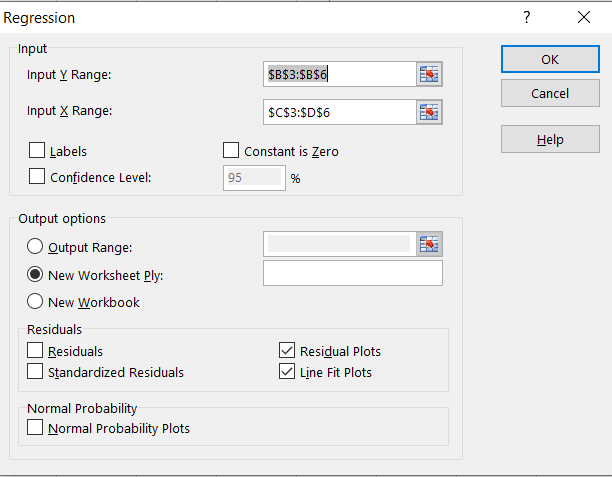The multiple regression would look something like this: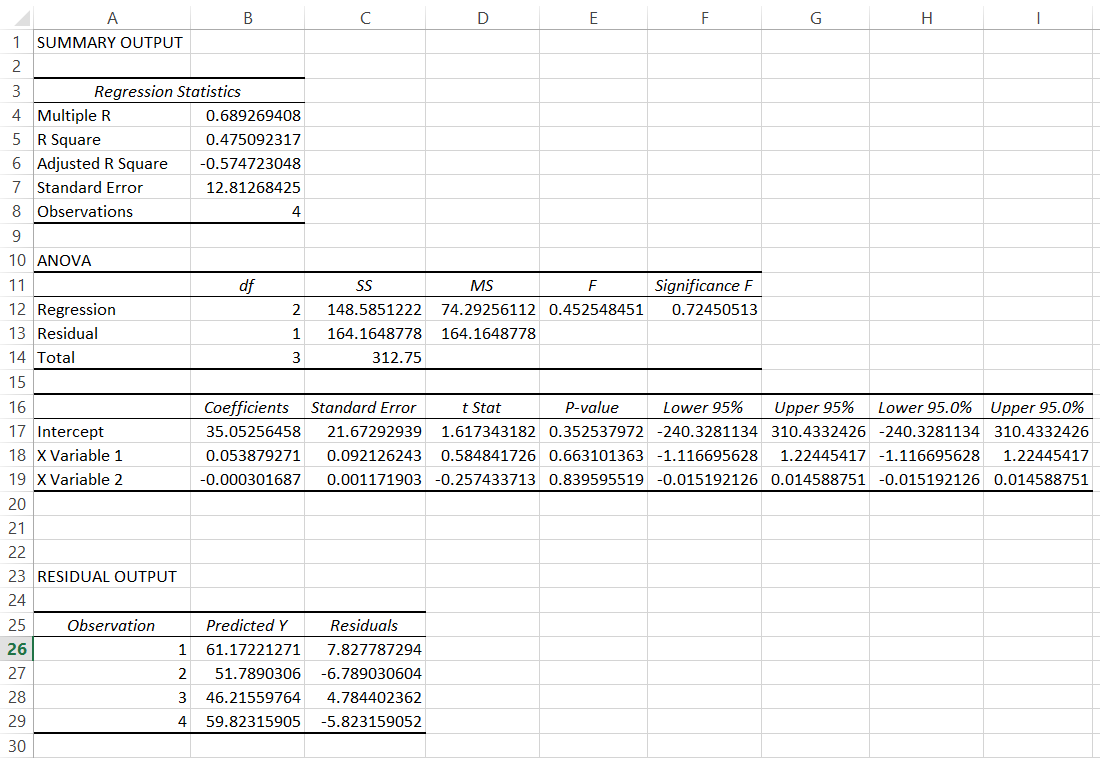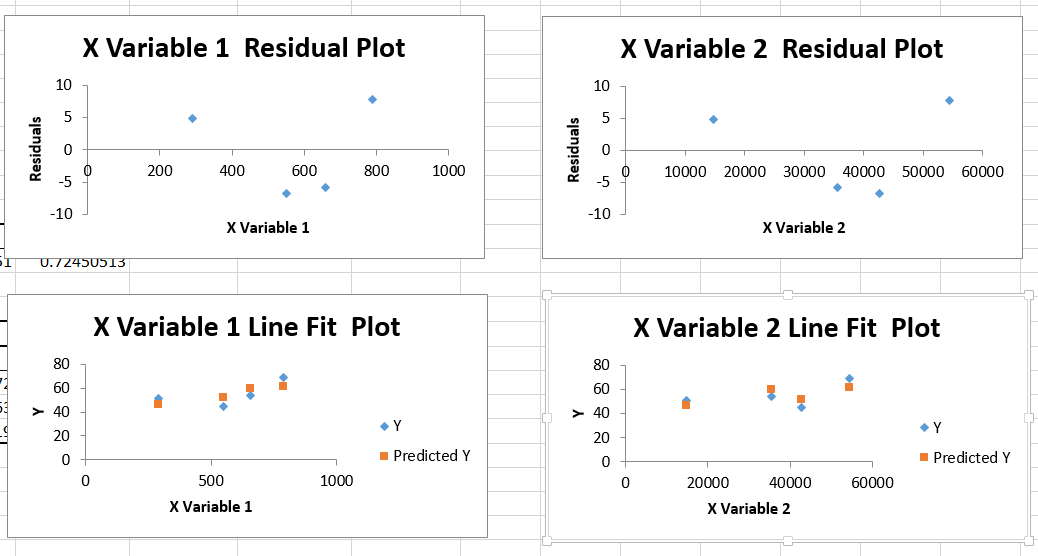### Interpreting the results

#### Regression Statistics

• Multiple R (aka. Correlation Coefficient) is higher than 0. It means that there is a positive relationship. The higher the number (the closer to 1) the stronger the relationship.
• R square (aka. Coefficient of Determination) is equal to 0.47. That means 47% of values fit to the model of our analysis.
• Adjusted R Square you should focus on that in the situation of having more than one x values.
• Standard error is very high. This is the information that I cannot be confident about the analysis too much because of the high error I need to remember about.
• Observations is not what need to be analyzed. This is the number of observations provided by me to the analysis.

#### Anova

• F is a F-test of the null hypothesis and the Significance F is a p-value of F.
• Significance F is the most important here. The first thing to note is that this number is much higher than alpha (0.5), meaning the observation is not statistically significant.
• Lower 95% and Upper 95% are the boundaries of confidence interval

#### Residual Output

• Predicted Y is the value of predicted data points. You should compare them with the atual ones.
• Residuals are telling us on how far from the predicted values the actual data points are.
• The graph on the right side of the screen shows the residual values and their fit to the model. In the example we’re talking about now, we don’t really care. However, due to the many regressions you do in business cases, it’s extremely important to adjust your data as much as possible. Even 1% of the variance can lead to wrong assumptions. Therefore, you should take care of the rest to keep them as close to zero as possible.

These are the basic steps for performing regression analysis in Excel using the Analysis ToolPak. By following these steps, you can easily model the relationship between a dependent variable and one or more independent variables and analyze the results of the regression.